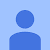# Part 1: What is Tensor?

A PyTorch tensor is nearly the same thing as a numpy array, but with an additional restriction which unlocks some additional capabilities. It’s the same in that it, too, is a multidimensional table of data, with all items of the same type. However, the restriction is that a tensor has to use a single basic numeric type for all components. As a result, a tensor is not as flexible as a genuine array of arrays, which allows jagged arrays, where the inner arrays could have different sizes. So a PyTorch tensor cannot be jagged. It is always a regularly shaped multidimensional rectangular structure.

If tensor is just an array, how can we know how this was created, how can we store its gradient? So, to solve this problem a wrapper around tensor was created. As a result, a tensor object has certain properties

• data (the tensor under the variable), `data.numpy()`
• requires_grad (the gradient computed for this variable, must be of the same shape and type of .data)
• device
• dtype
• pin_memory
• vector is of [1, N], and in pytorch it display its size as [N]
`abcd = torch.rand((3,3), requires_grad=True)print(abcd.grad)c = abcd.sum()c.backward()print(abcd.grad)Nonetensor([[1., 1., 1.],        [1., 1., 1.],        [1., 1., 1.]])`

# Part 2: Tensor generator

Generator

• `a=torch.rand((3,4))` or `a=torch.randn((3,4))` This will generate random floating tensor array.
• generate tensor from numpy.array
`import numpy as npa = np.ones(5)b = torch.from_numpy(a)a=torch.zeros((3,3))np = a.numpy()b = torch.from_numpy(np)`

(1) `from_numpy` indicates that the numpy array and tensor share the same memory

(2) `torch.tensor(np_array)` will generate a new tensor that does not share memory with numpy array

`a=np.ones((3,3))t = torch.from_numpy(a)print(t)a[0,0]=20print(t)tensor([[1., 1., 1.],        [1., 1., 1.],        [1., 1., 1.]], dtype=torch.float64)tensor([[20.,  1.,  1.],        [ 1.,  1.,  1.],        [ 1.,  1.,  1.]], dtype=torch.float64)`

(3) a.numpy() or a.data.numpy() is used to transform to numpy array

• scalar
`np_array[index].item()a=torch.rand((3,4))print(a)print(a[1,1].item())`
• list
`a=[1,2,3]b=torch.tensor(a)c=b.tolist()a = torch.Tensor(list(range(1,10)).view(3,3)`
• tensor
`1. torch.full((3,3), 2, dtype=float)2. torch.arange(2, 20, 3).view((2,3))3. torch.linspace(2, 20, 16).view(4,4)4. torch.normal(0, 3, size=(1,5))5. torch.randn((3,3)) # normal distribution with 0 mean and 1 std6. torch.rand((3,3)) # [0, 1]7. torch.randint(0, 10, (3,3))8. torch.randperm(30)`

# 3.1 Modify itself

• `fill_` or `normal_`
`a=torch.rand((3,4))a.fill_(3)print(a)a=torch.empty((3,4))a.normal_(std=3)print(a)`
• `resize_`
`a=torch.empty((3,4))a.resize_(1, 2, 2, 3)print(a.shape)`

this function is very similar to `view` The difference, however, is that `view` will not change original tensor.

`a=torch.empty((3,4))b = a.view((2,-1))c = a.view((2, 3, 2))print(a.shape)print(b.shape)print(c.shape)torch.Size([1, 2, 2, 3])torch.Size([2, 6])torch.Size([2, 3, 2])`

`torch.reshape` is very similar to `view`

• change data type via `type`
`a=torch.randn((3,4), dtype=float)print(a.dtype)b = a.type(torch.FloatTensor)print(b.dtype)torch.float64torch.float32`
• change the dimension of a tensor

Increase new dim via `unsqueeze`

`a=torch.randn((3,4), dtype=float)print(a.shape)b = a.unsqueeze(dim=2)print(b.shape)b = a.unsqueeze(dim=0)print(b.shape)torch.Size([3, 4])torch.Size([3, 4, 1])torch.Size([1, 3, 4])`

Use `cat` increase in existing dimensions

`x = torch.rand([11, 25])y = torch.rand([11, 1])z = torch.cat((x,y),axis=1)print(z.shape)`

Use `stack` increasing in non-existed dimensions

`x = torch.rand([11, 25])y = torch.rand([11, 25])z = torch.stack((x,y), dim=0)print(z.shape)torch.Size([2, 11, 25])`

Use `transpose` to change the dimension

`t = torch.rand(( 3, 100, 300))t_transpose = torch.transpose(t, 0, 2) t_transpose = torch.transpose(t_transpose, 0, 1) # c*h*w     h*w*c t shape:torch.Size([3, 100, 300]) t_transpose shape: torch.Size([100, 300, 3])`

# 3.2 Internal property

• sort the elements in the tensor
`a=torch.randn((3,4), dtype=float)print(a)top_p, top_class = a.topk(2, dim=1)print(top_p)print(top_class)tensor([[-0.3778, -2.2176,  2.7136, -0.7310],        [-1.6162, -0.9407,  0.9365,  0.9484],        [-0.7596, -0.0329, -0.6310, -0.9449]], dtype=torch.float64)tensor([[ 2.7136, -0.3778],        [ 0.9484,  0.9365],        [-0.0329, -0.6310]], dtype=torch.float64)tensor([[2, 0],        [3, 2],        [1, 2]])`

# Part 4: Tensor Calculation

Multiplication

`a=torch.randn((3,4))b=torch.randn((4,1))c = a@bprint(c)print(a)print(b)print(torch.matmul(a,b))`

In PyTorch, we have `torch.mm`, `torch.mul`, and `torch.matmul` , please check The difference between torch.mm, torch.mul, torch.matmul to see the difference.

• `torch.mul` point by point multiplication
• `torch.mm(a,b)` matrix multiplication for two-dimensional matrix
• `torch.matmul` high-dimensional matrix multiplication

`a=torch.ones((1,))print(a)print(id(a))a = a+torch.ones((1,))print(id(a))a +=torch.ones((1,))print(id(a))140626975119168 # a=a+ will change MEM address140626975120896 # a += will not change MEM address140626975120896`

# Reference

Tutorial

--

--

--

Love podcasts or audiobooks? Learn on the go with our new app.

## Some Challenging and Interesting Practical Points on SQL## Never Underestimate Time Complexity## Linux Boot Process — Part 1## Web Scraping in .NET C### Testing Swift code with SKProduct## Cypress Locators Strategy## Design system: How to efficiently scale development?## Software Engineer View — Cook the life## Implement ResNet with PyTorch## Introducing PyTorch-accelerated## A little thinking on avoiding GPU memory outage during the model training (PyTorch)